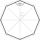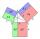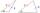# Inner angles

The inner angles of the triangle are 30°, 45° and 105° and its longest side is 10 cm. Calculate the length of the shortest side, write the result in cm up to two decimal places.

Result

a =  5.18 cm

#### Solution:Try calculation via our triangle calculator.

Leave us a comment of example and its solution (i.e. if it is still somewhat unclear...):

Showing 0 comments:Be the first to comment!#### To solve this example are needed these knowledge from mathematics:

Cosine rule uses trigonometric SAS triangle calculator. See also our trigonometric triangle calculator.

## Next similar examples:

1. Side cIn △ABC a=2, b=4 and ∠C=100°. Calculate length of the side c.
2. Triangle and its heightsCalculate the length of the sides of the triangle ABC, if va=5 cm, vb=7 cm and side b is 5 cm shorter than side a.
3. The mastA 40 m high mast is secured in half by eight ropes of 25 m long. The ends of the ropes are equidistant from each other. Calculate this distance.
4. SinesIn ▵ ABC, if sin(α)=0.5 and sin(β)=0.6 calculate sin(γ)
5. Centre of massThe vertices of triangle ABC are from the line p distances 3 cm, 4 cm and 8 cm. Calculate distance from the center of gravity of the triangle to line p.
6. TriangleCalculate the area of ​​the triangle ABC if b = c = 17 cm, R = 19 cm (R is the circumradius).
7. LawsFrom which law follows directly the validity of Pythagoras' theorem in the right triangle? ?
8. Two triangles SSATwo triangles can be formed with the given information. Use the Law of Sines to solve the triangles. A = 59°, a = 13, b = 14
9. Theorem proveWe want to prove the sentense: If the natural number n is divisible by six, then n is divisible by three. From what assumption we started?
10. TrigonometryIs true equality? ?
11. Three unknownsSolve the system of linear equations with three unknowns: A + B + C = 14 B - A - C = 4 2A - B + C = 0
12. Elimination methodSolve system of linear equations by elimination method: 5/2x + 3/5y= 4/15 1/2x + 2/5y= 2/15
13. AverageIf the average(arithmetic mean) of three numbers x,y,z is 50. What is the average of there numbers (3x +10), (3y +10), (3z+10) ?
14. AP - simpleDetermine the first nine elements of sequence if a10 = -1 and d = 4
15. PIN - codesHow many five-digit PIN - code can we create using the even numbers?
16. SequenceWrite the first 7 members of an arithmetic sequence: a1=-3, d=6.
17. BlocksThere are 9 interactive basic building blocks of an organization. How many two-blocks combinations are there?